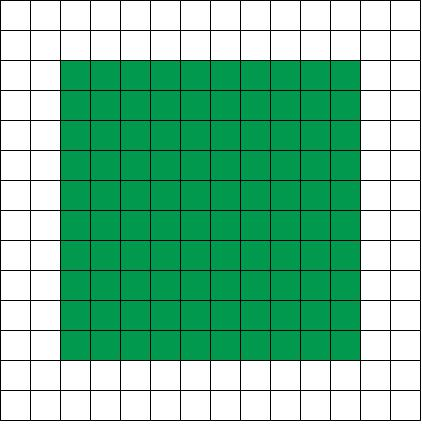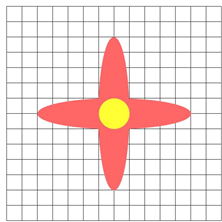# Rotational symmetry and transformations

## What is rotational symmetry

In its most basic explanation, rotation symmetry happens when a shape looks the same even after you rotate it. Of course, there's more mathematical terms and concepts associated with it, and we'll explore them below.

## Order of rotational symmetry

When you make a full rotation, the number of times the shape looks the same is called the order of rotational symmetry. If a shape has order 4 rotational symmetry, it means that when you rotate the shape all the way around till it lands back onto its original position, there's 4 times in which the shape looks the same.

## How to find the center of rotation

The center of rotation is the center point that you rotate the shape from. When carrying out rotational symmetry, you should always be rotating the shape from its center of rotation, rather than a point other than the center.

## Angle of rotation

The smallest angle that obtains the first shape that has rotational symmetry to the original shape is called the angle of rotation. An example is rotating a square shape. To do a full rotation, you'll be turning the square 360 degrees around. What is the angle of rotation? The smallest angle that you'll need to get the square to look like the original square again is 90 degrees! That's because there's four sides to a square and 360 divided by 4 is 90 degrees.

It's usually not the easiest to determine the angle just by looking at a shape, so we often use the order of rotational symmetry to describe rotational symmetry.

## Practice problems

Let's walk through rotational symmetry examples to explain the concepts we described above.

Question 1:

What are the number of lines of symmetry and the order of rotation for this image?

Solution:

In this question, we are looking for the number of lines of symmetry and the order of rotation of this shape:

First, we need to determine the centre of rotation symmetry. It's simple! The centre of rotation symmetry is right at the centre of the shape.

Now, we can draw the lines of symmetry on the shape. There are in fact 6 lines of symmetry. And we conclude that the order of rotation is also 6

Question 2:

For the shape below:

Where is the centre of rotation?

What are the order and the angle of rotation?

Show your answers in degrees and as fraction of a turn.

Solution:

For this question, the centre of rotation is just at the centre of the shape. We find that there are 4 lines of symmetry for the shape, and the order of rotation is 4.

Next, how do we find the angle of rotation? It's easy. We divide 360 degree by the order of rotation. So, for this example we divide 360 degree by 4 = 90 degree

This example also asks you to show the angle of rotation as fraction. To turn angle of rotation into fraction, we divide the angle of rotation by 360 degree. So, we divide 90 degree by 360 degree $= \frac{1}{4}$

Test yourself with this short quiz to see if you're able to visually spot rotational symmetry.

You'll soon realize that rotational symmetry has a lot to do with tessellations. Tessellations use translations and also reflections, but it could also require dealing with rotations. Feel free to check out the linked related chapters to learn how to make a tessellation after you're confident with rotational symmetry!

### Rotational symmetry and transformations

When an object is turned around its center of rotation to certain degrees and the object looks the same, we can say it has rotational symmetry. We will learn the details of rotational symmetry including, lines of symmetry, order of rotation, and angle of rotation.

#### Lessons

• Introduction
Types of transformation:
What are translations, reflections and rotations?
a)
1. What is line symmetry?
2. What is rotational symmetry?

b)
Types of transformation:
What are translations, reflections and rotations?

• 1.
All of the following figures have rotation symmetry.
• Where is the centre of rotation?
• What are the order and the angle of rotation? Show your answers in degrees and as fraction of a turn.
a)b)c)• 2.
What are the number of lines of symmetry and the order of rotation?
a)b)